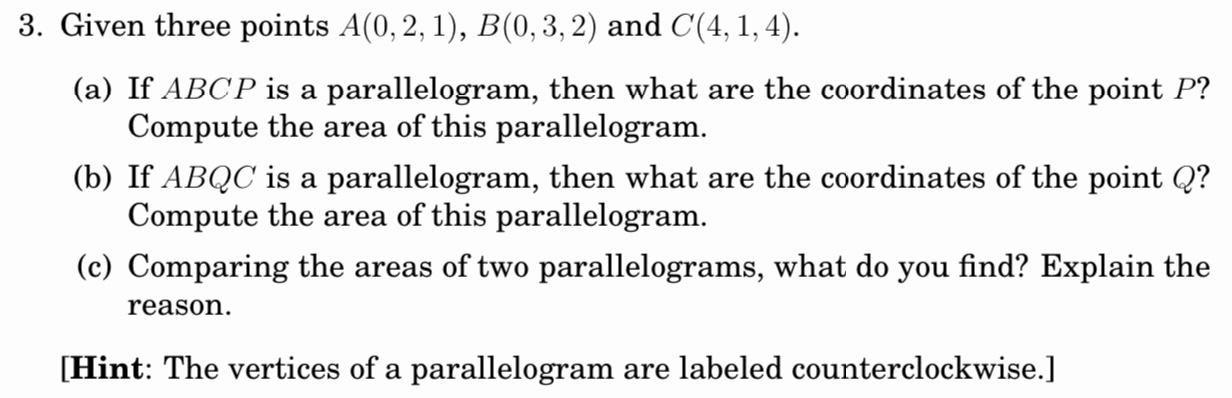Home / Answered Questions / Other / 3-given-three-points-a-0-2-1-b-0-3-2-and-c-4-1-4-a-if-abcp-is-a-parallelogram-then-what-are-the-coor-aw589

# (Solved): 3. Given Three Points A(0, 2, 1), B(0,3, 2) And C(4, 1, 4). (a) If ABCP Is A Parallelogram, Then Wha...3. Given three points A(0, 2, 1), B(0,3, 2) and C(4, 1, 4). (a) If ABCP is a parallelogram, then what are the coordinates of the point P? Compute the area of this parallelogram. (b) If ABQC is a parallelogram, then what are the coordinates of the point Q? Compute the area of this parallelogram. (c) Comparing the areas of two parallelograms, what do you find? Explain the reason. [Hint: The vertices of a parallelogram are labeled counterclockwise.]

We have an Answer from Expert